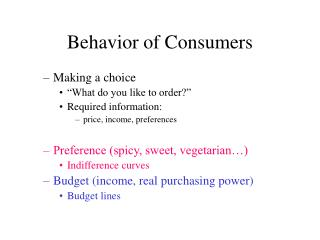# Behavior of Consumers - PowerPoint PPT PresentationDownload PresentationBehavior of Consumers

Behavior of ConsumersDownload Presentation## Behavior of Consumers

- - - - - - - - - - - - - - - - - - - - - - - - - - - E N D - - - - - - - - - - - - - - - - - - - - - - - - - - -
##### Presentation Transcript

1. Behavior of Consumers • Making a choice • “What do you like to order?” • Required information: • price, income, preferences • Preference (spicy, sweet, vegetarian…) • Indifference curves • Budget (income, real purchasing power) • Budget lines

2. What do you like to have if you can afford anyway? Pizzas vs Beers “Basket”: (#pizza, #beer) (x,y) HL stands for the happiness level. Characterize the Preference beer x x x x x x x x (High HL) x x (Low HL) pizza

3. Indifference curve: A collection of baskets, all of which the consumer considers equally desirable. An indifference curve usually slopes downward. More is better --- the higher indifference curves, the better. Indifference curve beer HL4 HL3 HL2 HL1 pizza

4. In general, the indifference curves fill the entire x-y plane. Any two indifference curves never cross each other. Relationships among indifference curves beer B C A HL4 HL3 HL2 HL1 pizza

5. Draw indifference curves for blue pens and black pens (assume that they are equally desirable). Draw your indifference curves for coffee and coffee mates (assuming that you must have two coffee mates for each cup of coffee) Important examples

6. Suppose one of your indifference curves goes through two baskets, (7, 2) and (6, 5). You currently have (7, 2). Which trade would you like to accept? “I’ll give you four beers to exchange for a pizza.” “I’ll give you two beers for a pizza.” “I’ll give you three beers for a pizza.” Introduce the concept of marginal value beer * 5 * 2 A pizza 6 7

7. Marginal value • The 7th pizza is worth exactly 3 beers. • “the marginal value of the 7th pizza equals 3 beers.” • Def: The marginal value of X in term of Y is the units of Y for which the consumer would be just willing to trade on one unit of X.

8. If you would like to exchange two bears for a pizza, the marginal value of that pizza in your mind equals two beers. the marginal value of a pizza at the basket (7,2) = the slope of IC at (7,2) The steeper the indifference curve, the greater the marginal value of a pizza. Marginal value as a slope of an indifference curve beer Indifference Curve (IC) tangent line A 2 7 pizza

9. What is the marginal value of dime in terms of nickels? What about the case of coffee-coffee mates? Example

10. Why? A: A starving person who have many bottles of beers would not like beers. B: A thirsty person who have many pieces of pizzas would not like pizzas. 5. Indifference curves are usuallyconvex. beer A B pizza

11. Summarize the properties of IC: • Use indifference curves to describe preference: • a collection of baskets all of which you consider equally desirable • Properties of well-behaved IC: • Slope downward (usually true) • More is better (usually true) • Never cross (always true) • Fill the entire plane (usually true) • Convex (usually true) • Slope of the indifference curve • Indicate the subjective value of x in terms of y; • ‘Marginal value of x in terms of y’

12. Exceptions Properties of well-behaved IC: • Slope downward (usually true) Exception- coffee/coffee mates • More is better (usually true); Exception- coffee/coffee mates • Never cross (always true) – no exceptions • Fill the entire plane (usually true) Exception- discrete commodity space • Convex (usually true) Exception- nickels/dimes; coffee/coffee mates